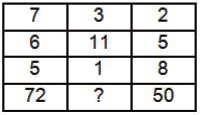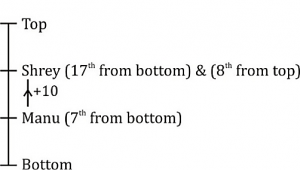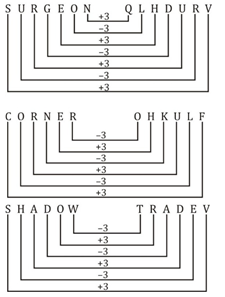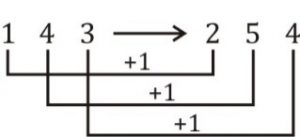Latest SSC jobs   »   Reasoning Quiz [beginner level] For SSC...

# Reasoning Quiz [beginner level] For SSC CGL : 1st January

Q1. In a row of people Manu is 7th from bottom end of row. Shrey is 10 ranks above Manu. If Shrey is 8th from top, then how many people are there in this row?
(a) 25
(b) 26
(c) 24
(d) 23

Direction (2-3): In the following question, select the word which cannot be formed using the letters of the given word.

Q2. Instructor
(a) Rust
(b) Tort
(c) Stop
(d) Stint

Q3. Encourage
(a) Courage
(b) Court
(c) Race
(d) Encore

Q4. In a certain code language, “SURGEON” is written as “QLHDURV” and “CORNER” is written as “OHKULF”. How is “SHADOW” written in that code language?
(a) DRTERS
(c) UQBCFU
(d) TFBCPX

Q5. In a certain code language, “SUN” is written as “54” and “PUT” is written as “57”.
How is “CAT” written in that code language?
(a) 28
(b) 24
(c) 52
(d) 36

Q6. In the following question, by using which mathematical operators will the expression become correct?
14 ?2 ?4 ?6 ? 4
(a) ×, ÷, > and ×
(b) ÷, ×, > and ×
(c) ÷, +, = and ×
(d) ÷, +, > and ×

Q7. In the following question, correct the equation by interchanging two signs.
43 + 9 – 6 ÷ 3 × 8 = 50
(a) ÷ and ×
(b) + and ÷
(c) – and +
(d) – and ×

Q8. If 4 * 9 % 2 = 47 and 9 * 0 % 6 = 84, then 5 * 3 % 7 = ?
(a) 38
(b) 51
(c) 42
(d) 46

Q9. If 1/4/3 = 254 and 3/6/8 = 479, then 5/2/7 = ?
(a) 416
(b) 461
(c) 368
(d) 638

Direction (10): In the following question, select the number which can be placed at the sign of question mark (?) from the given alternatives.
Q10.(a) 38
(b) 40
(c) 42
(d) 44

#### Solutions:

S1. Ans. (c);
Sol.∴ Total people in this row = 8 + 17 – 1 = 24

S2. Ans. (c);
Sol.
‘STOP’; there is no ‘P’ in the given word.

S3. Ans. (b);
Sol.
‘COURT’; There is no ‘T’ in the given word.

S4. Ans. (b);
Sol.S5. Ans.
Sol.
(b); SUM = 19 + 21 + 14 = 54
PUT = 16 + 21 + 20 = 57
CAT = 3 + 1 + 20 = 24

S6. Ans. (b);
Sol.
14 ÷ 2 × 4 > 6 × 4
7 × 4 > 24
28 > 24

S7. Ans. (c);
Sol.
43 + 9 – 6 ÷ 3 × 8 = 50
43 – 9 + 6 ÷ 3 × 8 = 50
43 – 9 + 2 × 8 = 50
43 – 9 + 16 = 50
59 – 9 = 50
50 = 50

S8. Ans.(d);
Sol.
49 – 2 = 47
90 – 6 = 84
53 – 7 = 46

S9. (d);
Sol.Similarly 368 → 479
527 → 638

S10. (d);
Sol.
7 × 6 + 6 × 5 = 42 + 30 = 72
3 × 11 + 11 × 1 = 33 + 11 = 44
2 × 5 + 5 × 8 = 10 + 40 = 50

Click here for best SSC CGL mock tests, video course, live batches, books or eBooks

#### Congratulations!General Awareness & Science Capsule PDF